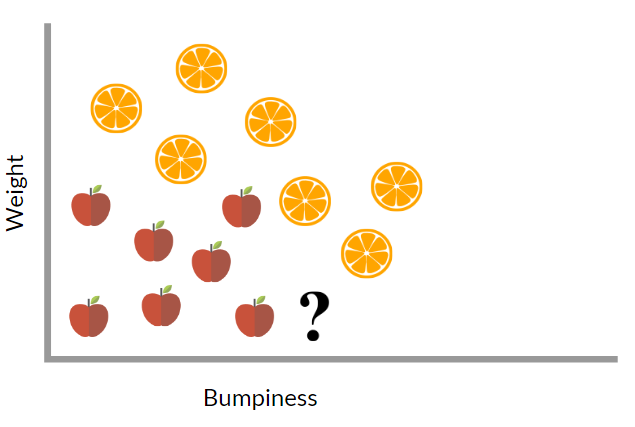# Posts taggedMachine Learning

## Deep Learning: A Brief Introduction

Deep Learning is the use of “deep” Neural Networks as a tool in Machine Learning. (If you are unsure of what ML is please see my previous post)

This type of approach (as the name implies) is loosely modeled after human neurology. Let’s start by examining one of the simple problems for humans, but up until a few years ago was nearly impossible for a computer to do with any reasonable accuracy.

Recognizing handwritten digits may seem simple at first, but writing code to tell the difference between a 3 and 5 isn’t as simple as: if(x!=y)

Neural networks are a set of algorithms, modeled loosely after the human brain, that are designed to recognize patterns.

**These patterns are the similarity between all the 3s**

There are 3 main parts to this neural network, the input layer, the hidden layer(s), and the output layer.

The Input Layer in which the original image is ‘inputted’ into the network. There are two hidden layers that help process the image and the final layer which makes the final decision.

The first layer takes in the images as a vector of pixels: see the animation below.

Then (in theory) the next layer looks for edges by weighting the pixel values, in order to have a higher value if an edge exists, and a lower value if that edge doesn’t exist.

This is done by having a negative value for w for all pixels where no pixels should exist if there was an edge, and positive value pixels where a pixel should exist if there were are an edge there. This weighted sum would give a positive value if an edge was present and a negative value is no edge was detected.

This weighted sum could fall anywhere on the number line. We would like these sums to be a value between 0 and 1. In order to be sure only the presence of an edge or not is the only thing affecting the next layer, we pass the weighted sum through an Activation Function.

A similar process is done for the following layers, until the final layer where we normalize the values into probabilities of each number from zero through nine.

## Machine Learning: A Brief Introduction

Whether it be through search, voice assistant, or spam filtering, the vast majority of people with an internet connection have interacted in some way with Artificial Intelligence. In fact, many leaders in the tech industry – including Elon Musk and others –  have expressed their concerns about the negative consequences of AI.

In order to truly understand what AI is, let’s start by defining it. Merriam Webster defines AI as the capability of a machine to imitate intelligent human behavior. That manifests itself in an infinite number of ways, including everything from spam detection, self-driving cars, agriculture, to cancer research.

Today, the most prominent region in AI is Machine Learning (ML). Machine Learning provides systems the ability to automatically learn and improve from experience without being explicitly programmed. In essence, Machine Learning allows machines to ‘learn’ from previous examples.

With this type of approach, there are many types of problems we can tackle, one of which is classification. Imagine we needed to tell whether a fruit was an apple or an orange exclusively based on its relative bumpy-ness and its weight.

We would start by plotting several examples of apples and oranges on a graph, with one axis for bumpy-ness and one axis for weight.Given the relative bumpy-ness and weight of fruit, there are multiple ways we could assume the identity of the fruit.

The simplest of the methods is called K-Nearest Neighbor; we would compute the closest fruit to the mystery fruit, (ie: using the Pythagorean distance) and assume that it belongs in the same category. Another method is the Logistic Regression; this is were a decision boundary is drawn and the fruit classified based on the boundary line.

In the figure above we can see that in both cases (since the mystery fruit is both below the decision boundary and closest to an apple) it is fairly evident that the mystery fruit is most likely an apple.

There are many other ways to solve this problem, including Support Vector Machines, decision trees, and similar algorithms.

In my next post, I hope to discuss what is considered the most successful of Machine Learning Algorithms: Deep Neural Networks aka Deep Learning.